# IBPS Clerk Mains 2017 – Reasoning DAY 3

Dear Banking Aspirants,

IBPS CLERK Mains 2017, the last exam of the year 2017 is going to held in 21.01.2018.There is only few days in our hands for preparation and you have to work hard to achieve your goal, because the competition is very high as per the vacancy is concerned.

So start your preparation now with this study Planner for IBPS Clerk Mains. It’s a 20 days complete planner with various Aptitude, Reasoning, English, Current affairs & Static Gk topics Included. There are 4 Mock tests available in this planner.

We have planned this Planner in Such a way that this will cover the entire current affairs quiz from the month of October to January (1st week). Because Banking Awareness, Current Affairs, Static Gk & Computer Awareness will play a vital role in cracking the IBPS Clerk Mains exam. Though the exam is getting tougher and tougher for each and every Year, Only consistently hard working candidates will crack the exam. So be a continuous hard worker and grab the opportunity to achieve your success.

## Try Try untill you succeed!!!!!!!!!!!

So as per the planner here is content related to Reasoning .

IBPS CLERK Mains 2017-Reasoning Day 3

Reasoning

Time: 20 Minutes

Topic: Direction & distance

### Direction & Distance

D.1-3) Study the following information carefully and answer the following questions.

Two persons A and B are facing each other and the distance between A and B is 10 meter. Person A is facing either North or East. A and B are turned right and then walked 5 meters to reach Point C and Point D respectively. From Point C he turned 135 degree clockwise and walked 11 meters to reach point E. From Point D he turned left and walked 10 meters to reach Point F. Point F is west of starting point of A.

Q.1) If Point G is 5 meter east of Point F then Point G is in which direction with respect to Point D?

a) North-East

b) North-West

c) South-East

d) South-West

e) None of these

Q.2) If Point H is 8 meter south of B’s starting point then Point H is in which direction with respect Point F?

a) North-East

b) North-West

c) South-East

d) South-West

e) None of these

Q.3) Point F is in which direction with respect to starting point of B?

a) North-East

b) North-West

c) South-East

d) South-West

e) None of these

D.4-5) Study the following information carefully and answer the given questions.

P, Q, R, T and U are five friends standing in a football playground. P is in the middle of the ground and faces north. P kicks the ball in north east direction and R is the only person to receive the ball. Q is west of R and Q, P are form a straight line. R is related to P in the same way P is related to T who faces north. U is east of T but not in the line of Q and P.

Q.4) If the distance between Q and R is four meter and the distance between Q and P is two times of Q and R then what is the shortest distance between P and R (approximately)?

a) 7 meter

b) 8 meter

c) 9 meter

d) 11 meter

e) None of these

Q.5) If U is south of R then Q is in which direction with respect U?

a) North-East

b) North-West

c) South-East

d) South-West

e) None of these

Q.6) A man walks 5m towards north and then turn to his left and walks 10m.Then he turn to his right and walks 4m.In which direction is the man now from the starting point?

a) North East

b) North West

c) South East

d) South West

e) None of these

Q.7) Manoj walks 10m towards east and he turn to his right and walks 4m.Then he turn to his left and walks 5m. Finally he turn to his left and walks 4m.How far and in which direction is he from the starting point?

a) 15m, East

b) 15m, South

c) 15m, North

d) 15m, West

e) None of these

Q.8) Mohan starts from his house and walks 3m towards North-East and then turn to his left and walks 5m.Now, How far and in which direction is he from the starting point?

a) 4m, South

b) 4m, North

c) 4m, East

d) 4m, West

e) None of these

Q.9) Point A is 5m to the south of Point B. Point C is 3m to the east of Point B. Point F is 2m to the south of Point C. Point D is 5m to the west of Point F. Point E is 3m to the south of Point D. Point E is in which direction with respect to Point B?

a) North East

b) North West

c) South East

d) South West

e) None of these

Q.10) A man walks 5m towards south and turn to his right and walks 4m.Then he turns 900  in anti clockwise direction and walks 5m.How far and in which direction is he from the starting point, if he turns 900  clockwise direction instead of taking 900  anti clockwise direction?

a) 4m, East

b) 4m, West

c) 4m, North

d) 4m, South

e) None of these

D.11-13) Study the following information and answer the questions given below:

‘A&B’ means ‘Point A is 4m north of Point B’

‘A@B’ means ‘Point A is 7m south of Point B’

‘A^B’ means ‘Point A is 10m west of point B’

‘A\$B’ means ‘Point A is 3m east of point B’

Q.11)  If Q@W&R^T\$Y, then in which direction is Point Q with respect to Point Y?

a) West

b) North West

c) South West

d) South East

e) None of these

Q.12) If H\$J@K\$L^G, then how far and in which direction is Point K with respect to Point G?

a) 6m East

b) 7m West

c) 4m East

d) 1m West

e) None of these

Q.13) If T&Y^U@O&P, then which of the following points lies in a straight line?

a) OTY

b) TYP

c) PTU

d) POU

e) None of these

D.14-15) Study the following information and answer the questions given below:

Point A is 5m to the east of Point B. Point C is 10m to the North of Point D. Point D is 3m to the west of Point E. Point E is 2m to the South of Point F. Point B is 7m to the North of Point C.

Q.14) If Point F is 3m to the east of Point H, then what is the distance between Point H and B?

a) 17m

b) 15m

c) 12m

d) 10m

e) None of these

Q.15) In which direction is Point A with respect to Point D?

a) North West

b) South West

c) North East

d) South East

e) None of these

D.16-18) Study the following information and answer the questions given below:

Point I is to the south west of Point U, which is to the south of Point J. Point R, B and I lies in a straight line. Point U is 5m to the east of Point B. The distance between Point B and Point I is one-third of the distance between Point B and Point R. The distance between Point J and Point U is same as the distance between Point B and point R. The distance between Point J and Point U is twice the distance between Point J and Point T. The distance between Point B and Point I is twice the distance between Point B and Point U. Point R is to the north of Point B. Point J is to the west of Point T.

Q.16) How far and in which direction is Point R with respect to Point I?

a) 40m, North

b) 40m, South

c) 30m, West

d) 40m, East

e) None of these

Q.17) Which of the following points lies in a straight line?

a) JRT

b) BUI

c) UJT

d) TBR

e) None of these

Q.18) If the distance between Point J and Point U is six times the distance between Point Q and Point U. Point U is to the west of Point Q, then how far and in which direction is Point I with respect to Point Q?

a) 10√2m, South West

b) 10√2m, North West

c) 10√2m, South

d) 10√2m, South East

e) None of these

D.19-20) Study the following information and answer the questions given below:

Two persons Kavitha and Radha start walking from the same point. Kavitha walks 5m towards north and turn to his right and walks 12m, then she turn to his left and walk 5m and reach the school. Radha walks 8m towards south and turn to his left and walks 7m, then again she turn to his left and walks 8m to reach the library.

Q.19) How far and in which direction is Kavitha from the starting point with respect to library?

a) 7m North

b) 7m South

c) 7m West

d) 7m East

e) None of these

Q.20) In which direction is School with respect to library?

a) North West

b) South West

c) South East

d) North East

e) None of these

D.1-3)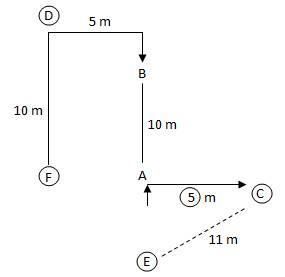1. (c)
2. (a)
3. (d)

D.4-5)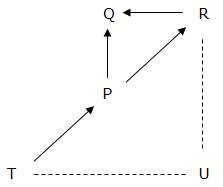1. (c)
2. (b)
3. (b)

North-west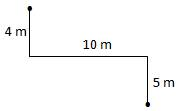1. (a)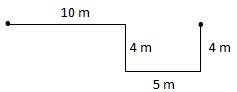8.(a)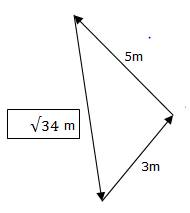1. (d)

South west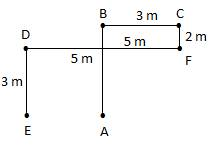1. (b)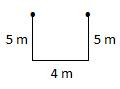4 m, west

11.(c)

Q @ W & R ^ T \$ Y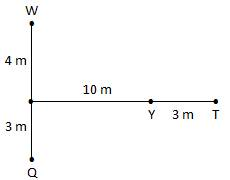1. (b)

H \$ J @ K \$ L ^ G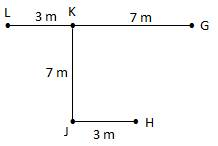1. (d)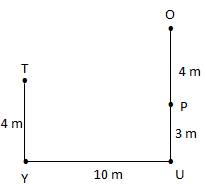D.14-15)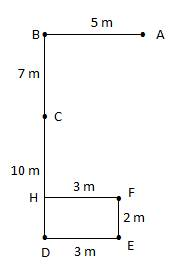1. (b)
2. (c)

D.16-18)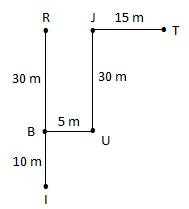1. (a)

40 m, north

1. (a)

JRT lies in a straight line

1. (a)

JV = 6 × QU

30 = 6 × QU

QU = 5 m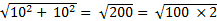= 10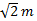, south west

D.19-20)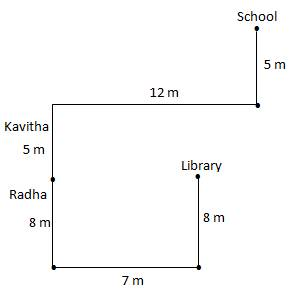1. (c)
2. (d)